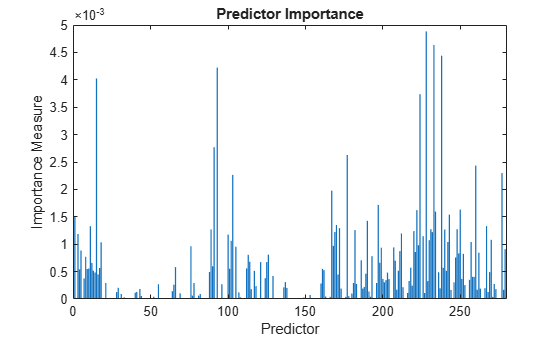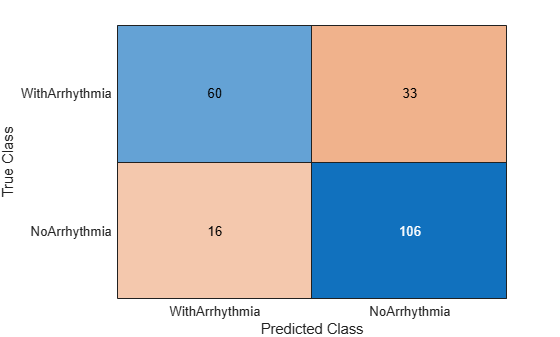# loss

Classification loss for classification ensemble model

## Syntax

``L = loss(ens,tbl,ResponseVarName)``
``L = loss(ens,tbl,Y)``
``L = loss(ens,X,Y)``
``L = loss(___,Name=Value)``

## Description

````L = loss(ens,tbl,ResponseVarName)` returns the Classification Loss `L` for the trained classification ensemble model `ens` using the predictor data in table `tbl` and the true class labels in `tbl.ResponseVarName`. The interpretation of `L` depends on the loss function (`LossFun`) and weighting scheme (`Weights`). In general, better classifiers yield smaller classification loss values. The default `LossFun` value is `"classiferror"` (misclassification rate in decimal).```
````L = loss(ens,tbl,Y)` uses the predictor data in table `tbl` and the true class labels in `Y`.```

example

````L = loss(ens,X,Y)` uses the predictor data in matrix `X` and the true class labels in `Y`.```

example

````L = loss(___,Name=Value)` specifies options using one or more name-value arguments in addition to any of the input argument combinations in the previous syntaxes. For example, you can specify the indices of weak learners in the ensemble to use for calculating loss, specify a classification loss function, and perform computations in parallel.```

Note

If the predictor data `X` or the predictor variables in `tbl` contain any missing values, the `loss` function might return NaN. For more details, see loss might return NaN for predictor data with missing values.

## Input Arguments

expand all

Full classification ensemble model, specified as a `ClassificationEnsemble` model object trained with `fitcensemble`, or a `CompactClassificationEnsemble` model object created with `compact`.

Sample data, specified as a table. Each row of `tbl` corresponds to one observation, and each column corresponds to one predictor variable. `tbl` must contain all of the predictors used to train the model. Multicolumn variables and cell arrays other than cell arrays of character vectors are not allowed.

If you trained `ens` using sample data contained in a table, then the input data for `loss` must also be in a table.

Data Types: `table`

Response variable name, specified as the name of a variable in `tbl`. If `tbl` contains the response variable used to train `ens`, then you do not need to specify `ResponseVarName`.

If you specify `ResponseVarName`, you must specify it as a character vector or string scalar. For example, if the response variable `Y` is stored as `tbl.Y`, then specify it as `"Y"`. Otherwise, the software treats all columns of `tbl`, including `Y`, as predictors.

The response variable must be a categorical, character, or string array, a logical or numeric vector, or a cell array of character vectors. If the response variable is a character array, then each element must correspond to one row of the array.

Data Types: `char` | `string`

Class labels, specified as a categorical, character, or string array, a logical or numeric vector, or a cell array of character vectors. `Y` must have the same data type as `tbl` or `X`. (The software treats string arrays as cell arrays of character vectors.)

`Y` must be of the same type as the classification used to train `ens`, and its number of elements must equal the number of rows of `tbl` or `X`.

Data Types: `categorical` | `char` | `string` | `logical` | `single` | `double` | `cell`

Predictor data, specified as a numeric matrix.

Each row of `X` corresponds to one observation, and each column corresponds to one variable. The variables in the columns of `X` must be the same as the variables used to train `ens`.

The number of rows in `X` must equal the number of rows in `Y`.

If you trained `ens` using sample data contained in a matrix, then the input data for `loss` must also be in a matrix.

Data Types: `double` | `single`

### Name-Value Arguments

Specify optional pairs of arguments as `Name1=Value1,...,NameN=ValueN`, where `Name` is the argument name and `Value` is the corresponding value. Name-value arguments must appear after other arguments, but the order of the pairs does not matter.

Before R2021a, use commas to separate each name and value, and enclose `Name` in quotes.

Example: `loss(ens,X,LossFun="exponential",UseParallel=true)` specifies to use an exponential loss function, and to run in parallel.

Indices of weak learners in the ensemble to use in `loss`, specified as a vector of positive integers in the range [1:`ens.NumTrained`]. By default, all learners are used.

Example: `Learners=[1 2 4]`

Data Types: `single` | `double`

Loss function, specified as a built-in loss function name or a function handle.

The following table describes the values for the built-in loss functions.

ValueDescription
`"binodeviance"`Binomial deviance
`"classifcost"`Observed misclassification cost
`"classiferror"`Misclassified rate in decimal
`"exponential"`Exponential loss
`"hinge"`Hinge loss
`"logit"`Logistic loss
`"mincost"`Minimal expected misclassification cost (for classification scores that are posterior probabilities)
`"quadratic"`Quadratic loss

• `"mincost"` is appropriate for classification scores that are posterior probabilities.

• Bagged and subspace ensembles return posterior probabilities by default (`ens.Method` is `"Bag"` or `"Subspace"`).

• To use posterior probabilities as classification scores when the ensemble method is `"AdaBoostM1"`, `"AdaBoostM2"`, `"GentleBoost"`, or `"LogitBoost"`, you must specify the double-logit score transform by entering the following:

`ens.ScoreTransform = "doublelogit";`

• For all other ensemble methods, the software does not support posterior probabilities as classification scores.

You can specify your own function using function handle notation. Suppose that `n` is the number of observations in `X`, and `K` is the number of distinct classes (`numel(ens.ClassNames)`, where `ens` is the input model). Your function must have the signature

``lossvalue = lossfun(C,S,W,Cost)``
where:

• The output argument `lossvalue` is a scalar.

• You specify the function name (`lossfun`).

• `C` is an `n`-by-`K` logical matrix with rows indicating the class to which the corresponding observation belongs. The column order corresponds to the class order in `ens.ClassNames`.

Create `C` by setting `C(p,q) = 1`, if observation `p` is in class `q`, for each row. Set all other elements of row `p` to `0`.

• `S` is an `n`-by-`K` numeric matrix of classification scores. The column order corresponds to the class order in `ens.ClassNames`. `S` is a matrix of classification scores, similar to the output of `predict`.

• `W` is an `n`-by-1 numeric vector of observation weights. If you pass `W`, the software normalizes the weights to sum to `1`.

• `Cost` is a K-by-`K` numeric matrix of misclassification costs. For example, ```Cost = ones(K) - eye(K)``` specifies a cost of `0` for correct classification and `1` for misclassification.

For more details on loss functions, see Classification Loss.

Example: `LossFun="binodeviance"`

Example: `LossFun=@Lossfun`

Data Types: `char` | `string` | `function_handle`

Aggregation level for the output, specified as `"ensemble"`, `"individual"`, or `"cumulative"`.

ValueDescription
`"ensemble"`The output is a scalar value, the loss for the entire ensemble.
`"individual"`The output is a vector with one element per trained learner.
`"cumulative"`The output is a vector in which element `J` is obtained by using learners `1:J` from the input list of learners.

Example: `Mode="individual"`

Data Types: `char` | `string`

Option to use observations for learners, specified as a logical matrix of size `N`-by-`T`, where:

When `UseObsForLearner(i,j)` is `true` (default), learner `j` is used in predicting the class of row `i` of `X`.

Example: `UseObsForLearner=logical([1 1; 0 1; 1 0])`

Data Types: `logical matrix`

Flag to run in parallel, specified as a numeric or logical `1` (`true`) or `0` (`false`). If you specify `UseParallel=true`, the `loss` function executes `for`-loop iterations by using `parfor`. The loop runs in parallel when you have Parallel Computing Toolbox™.

Example: `UseParallel=true`

Data Types: `logical`

Observation weights, specified as a numeric vector or the name of a variable in `tbl`. If you supply weights, `loss` normalizes them so that the observation weights in each class sum to the prior probability of that class.

If you specify `Weights` as a numeric vector, the size of `Weights` must be equal to the number of observations in `X` or `tbl`. The software normalizes `Weights` to sum up to the value of the prior probability in the respective class.

If you specify `Weights` as the name of a variable in `tbl`, you must specify it as a character vector or string scalar. For example, if the weights are stored as `tbl.w`, then specify `Weights` as `"w"`. Otherwise, the software treats all columns of `tbl`, including `tbl.w`, as predictors.

Data Types: `single` | `double` | `char` | `string`

## Output Arguments

expand all

Classification loss, returned as a numeric scalar or numeric column vector.

• If `Mode` is `"ensemble"`, then `L` is a scalar value, the loss for the entire ensemble.

• If `Mode` is `"individual"`, then `L` is a vector with one element per trained learner.

• If `Mode` is `"cumulative"`, then `L` is a vector in which element `J` is obtained by using learners `1:J` from the input list of learners.

When computing the loss, the `loss` function normalizes the class probabilities in `ResponseVarName` or `Y` to the class probabilities used for training, which are stored in the `Prior` property of `ens`.

## Examples

expand all

`load fisheriris`

Train a classification ensemble of 100 decision trees using AdaBoostM2. Specify tree stumps as the weak learners.

```t = templateTree(MaxNumSplits=1); ens = fitcensemble(meas,species,Method="AdaBoostM2",Learners=t);```

Estimate the classification error of the model using the training observations.

`L = loss(ens,meas,species)`
```L = 0.0333 ```

Alternatively, if `ens` is not compact, then you can estimate the training-sample classification error by passing `ens` to `resubLoss`.

Create an ensemble of boosted trees and inspect the importance of each predictor. Using test data, assess the classification accuracy of the ensemble.

Load the arrhythmia data set. Determine the class representations in the data.

```load arrhythmia Y = categorical(Y); tabulate(Y)```
``` Value Count Percent 1 245 54.20% 2 44 9.73% 3 15 3.32% 4 15 3.32% 5 13 2.88% 6 25 5.53% 7 3 0.66% 8 2 0.44% 9 9 1.99% 10 50 11.06% 14 4 0.88% 15 5 1.11% 16 22 4.87% ```

The data set contains 16 classes, but not all classes are represented (for example, class 13). Most observations are classified as not having arrhythmia (class 1). The data set is highly discrete with imbalanced classes.

Combine all observations with arrhythmia (classes 2 through 15) into one class. Remove those observations with an unknown arrhythmia status (class 16) from the data set.

```idx = (Y ~= "16"); Y = Y(idx); X = X(idx,:); Y(Y ~= "1") = "WithArrhythmia"; Y(Y == "1") = "NoArrhythmia"; Y = removecats(Y);```

Create a partition that evenly splits the data into training and test sets.

```rng("default") % For reproducibility cvp = cvpartition(Y,"Holdout",0.5); idxTrain = training(cvp); idxTest = test(cvp);```

`cvp` is a cross-validation partition object that specifies the training and test sets.

Train an ensemble of 100 boosted classification trees using `AdaBoostM1`. Specify to use tree stumps as the weak learners. Also, because the data set contains missing values, specify to use surrogate splits.

```t = templateTree("MaxNumSplits",1,"Surrogate","on"); numTrees = 100; mdl = fitcensemble(X(idxTrain,:),Y(idxTrain),"Method","AdaBoostM1", ... "NumLearningCycles",numTrees,"Learners",t);```

`mdl` is a trained `ClassificationEnsemble` model.

Inspect the importance measure for each predictor.

```predImportance = predictorImportance(mdl); bar(predImportance) title("Predictor Importance") xlabel("Predictor") ylabel("Importance Measure")```Identify the top ten predictors in terms of their importance.

```[~,idxSort] = sort(predImportance,"descend"); idx10 = idxSort(1:10)```
```idx10 = 1×10 228 233 238 93 15 224 91 177 260 277 ```

Classify the test set observations. View the results using a confusion matrix. Blue values indicate correct classifications, and red values indicate misclassified observations.

```predictedValues = predict(mdl,X(idxTest,:)); confusionchart(Y(idxTest),predictedValues)```Compute the accuracy of the model on the test data.

```error = loss(mdl,X(idxTest,:),Y(idxTest), ... "LossFun","classiferror"); accuracy = 1 - error```
```accuracy = 0.7731 ```

`accuracy` estimates the fraction of correctly classified observations.